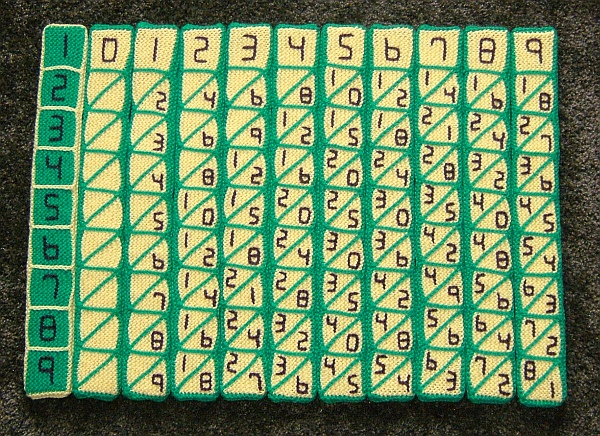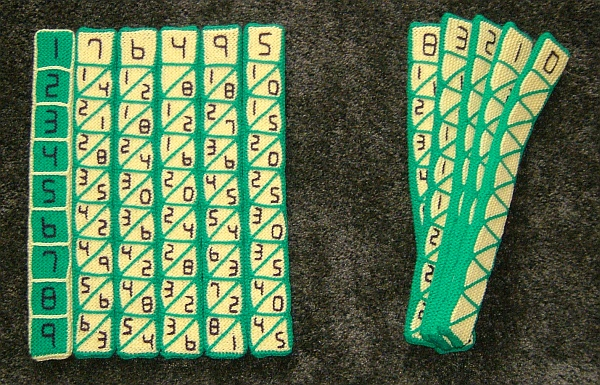### Math Monday: Knitting Napier’s Bones

by George Hart

Napier’s bones are a set of labeled sticks which John Napier invented in the 1600s for solving multiplication problems. Nowadays, you may be more likely to use a calculator, but if you want to multiply the old fashioned way, make yourself a set of Napier’s bones. You can use paper, wood, or other materials, but this set is knit. It is one of many mathematical knitting projects by Pat Ashforth, Steve Plummer, and Ben Ashforth.The image below shows how to arrange the bones if you wanted to multiply by 76495. For example, the bottom row, labeled 9 at left, allows you to read off 9 x 76495. The rightmost digit of the answer is the 5 seen in the triangle at right. Then read off the remaining digits by adding the two numbers in each parallelogram, carrying as necessary, e.g., 1+4 gives 5 as the next digit and 6+8=14 gives 4 as the following digit, with a carry of 1 into the digit after that. The result can be quickly read off as 688455.